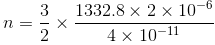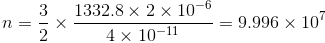NEET  >  Solved Examples: Kinetic Theory of Gases

# Solved Examples: Kinetic Theory of Gases Notes | Study NCERT Exemplar & Revision Notes for NEET - NEET

## Document Description: Solved Examples: Kinetic Theory of Gases for NEET 2022 is part of NCERT Exemplar & Revision Notes for NEET preparation. The notes and questions for Solved Examples: Kinetic Theory of Gases have been prepared according to the NEET exam syllabus. Information about Solved Examples: Kinetic Theory of Gases covers topics like and Solved Examples: Kinetic Theory of Gases Example, for NEET 2022 Exam. Find important definitions, questions, notes, meanings, examples, exercises and tests below for Solved Examples: Kinetic Theory of Gases.

Introduction of Solved Examples: Kinetic Theory of Gases in English is available as part of our NCERT Exemplar & Revision Notes for NEET for NEET & Solved Examples: Kinetic Theory of Gases in Hindi for NCERT Exemplar & Revision Notes for NEET course. Download more important topics related with notes, lectures and mock test series for NEET Exam by signing up for free. NEET: Solved Examples: Kinetic Theory of Gases Notes | Study NCERT Exemplar & Revision Notes for NEET - NEET
 1 Crore+ students have signed up on EduRev. Have you?

PROBLEM 1 (JEE MAIN):-
Calculate the number of molecules in 2 x 10-6 m3 of a perfect gas at 27°C and at a pressure of 0.01 m of mercury. Mean K.E of a molecule at 27°C = 4 x 10-11 J and g = 9.8 ms-2.
Solution:-
P = (1/3) (M/V) Cor PV = (1/3) MC2
But M = (m) (n)
Where ‘m’ is mass of one molecule and n is the number of molecules.
PV = (1/3) (m) C2
n = 3PV/mC2 = [3/2 PV] / [(1/2) mC2]
Here, P = 0.01 m of mercury = 0.01 x 13600 x 9.8Nm-2
V =  2 x 10-6 m3 = 1332.8 Nm-2
½ mc2 = 4 x 10-11JThus from the above observation we conclude that, the number of molecules would be 9.996 x 107.

Problem 2:-
You are throwing a birthday party and decide to fill the room with helium balloons. You also want to have a few larger balloons to put at the door. The smaller balloons are filled occupy 0.240 m3 when the pressure inside them is 0.038 atm and the temperature of the room is 70° F. What pressure should you fill the larger balloons to so that they occupy 0.400 m3?
Solution:-
The temperature of the room is assumed to be held constant, so it is extraneous information. Since you are dealing with volume and pressure, you would use Boyle's Law.
In accordance to data,
P1= 0.038 atm, V1= 0.240 m3
V2=0.400 m3
We have to find out V2.
Substitute the vale of P1, V1 and V2 in equation P1V1=P2V2
P1V1=P2V2
(0.038 atm)(0.240 m3) = P2(0.400 m3)
0.00912 = 0.400 P2             (units cancel out so that pressure will be in atm)
P2 = 0.0228 atm
From the above observation we conclude that, the pressure would be 0.0228 atm.

Problem 3:-
Find the RMS speed of a sample of neon gas at 80° F.
Solution:-
First convert Fahrenheit to Celsius.
°C = (°F- 32)/1.8
= (80 -32)/1.8
= 48/1.8
= 26.6
Convert Celsius to Kelvin.
K = °C + 273.15
= 26.6 + 273.15
= 299.75 K
Substitute the known information into the equation for RMS speed and solv, we get,.
vrms = √3RT/M
= √3(8.3144) (299.75)/ (20.179)
= 19.2 m/s
From the above observation we conclude that, the RMS speed of a sample of neon gas at 80° F would be 19.2 m/s.

The document Solved Examples: Kinetic Theory of Gases Notes | Study NCERT Exemplar & Revision Notes for NEET - NEET is a part of the NEET Course NCERT Exemplar & Revision Notes for NEET.
All you need of NEET at this link: NEET

## NCERT Exemplar & Revision Notes for NEET

291 docs
 Use Code STAYHOME200 and get INR 200 additional OFF

## NCERT Exemplar & Revision Notes for NEET

291 docs

### How to Prepare for NEET

Read our guide to prepare for NEET which is created by Toppers & the best Teachers

Track your progress, build streaks, highlight & save important lessons and more!

,

,

,

,

,

,

,

,

,

,

,

,

,

,

,

,

,

,

,

,

,

;